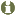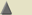# What is the rotational speed

 Introductions to the economic and social history of the 19th and 20th centuriesFISHERIAN TRAFFIC EQUATION

(up / ml) Fisher's equation of traffic or quantity equation of money bears the name of its developer Irving Fisher (American mathematician and economist, 1867-1947). It is:

M * v = Y * P

(M = money supply, v = speed of circulation of money per year, Y = real gross national product of an economy, P = price index of the gross national product)

The expression (Y * P) denotes the value of the entire annual product of an economy, which must be paid for with the available means of payment. This payment volume can be raised by (M * v), i.e. H. through the money supply M, which on average circulates v times in the economy every year. The rotational speed v cannot be measured directly, but is usually estimated using the equation v = Y * P / M.
If one assumes that v is constant, the price level changes (i.e. the inflation rate develops) according to the ratio in which the money supply M grows faster than the national income Y.© 2004 by Ulrich Pfister / Georg Fertig • mail: [email protected]